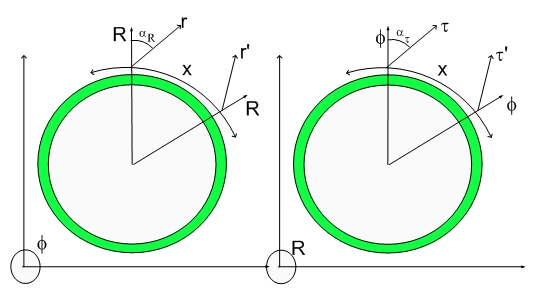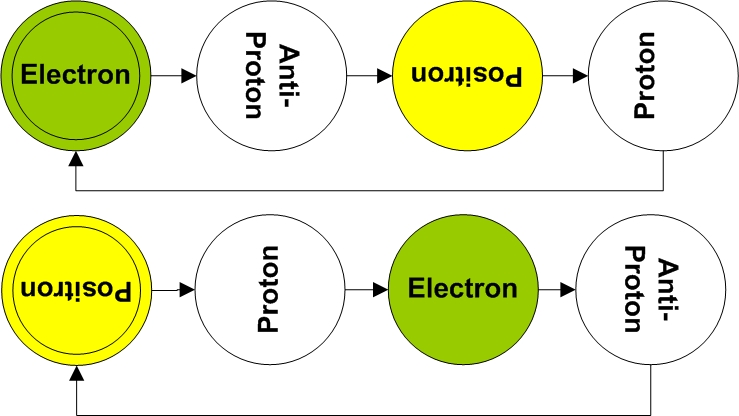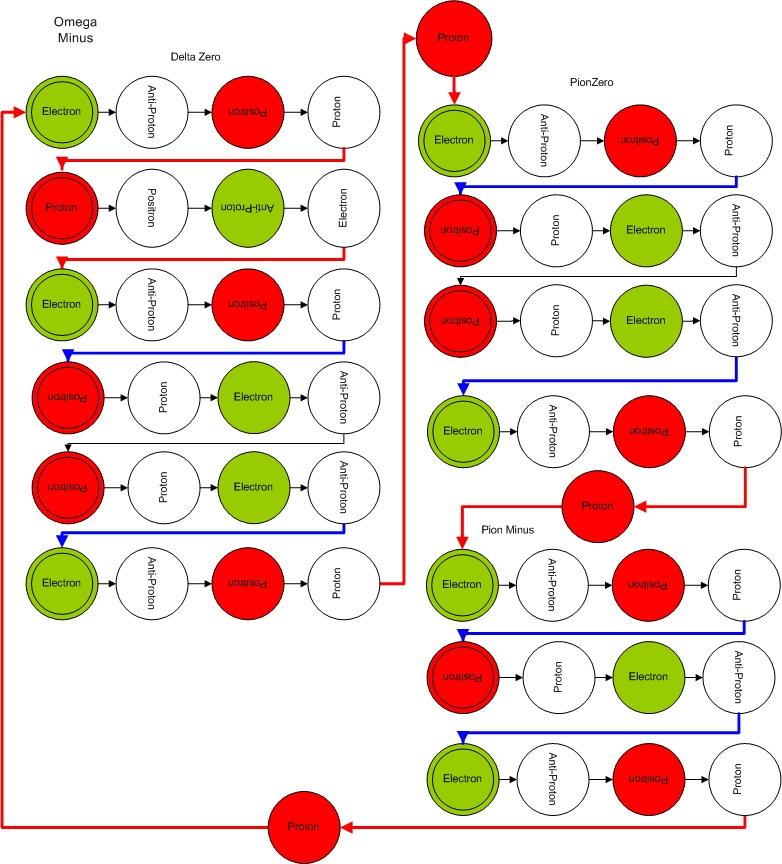Hypergeometrical Universe

Professor SmulskyProfessor Smulsky and General Relativity

Dear MP,

Thank you for your proposition to feel free to comment.

I. I begin from your words: "The physics is invisible to your eyes and instruments. It can only be seem with the eyes of the mind...", which pertain to the contemporary Physics. Such Physics is false science. It gives imaginary worlds instead the real one. I propose you to read my paper "Smulsky J.J. Conceptual Error in Contemporary Science // Proceedings of the Natural Philosophy Alliance. 13th Annual Conference 3-7 April 2006 at the University of Tulsa, OK, USA. Vol.3, No. 2. Published Space Time Analyses, Ltd. Arlington, MA,USA.- 2007. - Pp. 277-281." (http://hypergeo.s3.amazonaws.com/static/media/uploads/blog/CnErCS2.pdf)

II. Hypergeometrical Universe Theory, Grand Unification, inflation and other theories are based on the General Theory of Relativity (GTR). I send you my paper "Gravitation, field and rotation of Mercury perihelion", from which you will find that it is downfall of General Theory of Relativity. GTR can be forgotten and can be thrown out. Below I give some information about my paper:

Abstract: The basic components of a problem of rotation of Mercury perihelion are considered. It is shown, that offered by Paul Gerber the finite speed of gravity propagation has not substantiation. It is established, that with influence under the Newton law of gravity the perihelion in motionless reference system turns on 529.9" per one century, but on the data of observation it turns on 582.3" per one century. Early the rotation of the Sun on a movement of planets was not taken into account. The compound model of rotation of the Sun is offered which allows taking into account this influence. In view of rotation of the Sun the Newton law of gravity defines all size of perihelion rotation and defines all features of the planets movement.

Conclusions
1. The offered in 1898 by Paul Gerber the mechanism of finite speed of gravitation is
speculative and has no a substantiation.

2. The mechanism of finite speed of gravitation, based on analogy to electromagnetic influence, gives displacement of a perihelion 0.23" per one century, that more than in 200 times there is less than difference between observation and calculations under the Newton law of gravity.

3. The updated rate of perihelion rotation of Mercury relatively motionless space is 582.5" per one century, and by calculations at the Newton law of gravity it is 529.9" per one century, i.e. the difference between them is equal Δ'p0 = 52.6" per one century.

4. The finite speed of gravitation should result not only in change of displacement of
perihelion Δp0, but also two times of large changes of a cycle time ΔTtr and length of semiaxis a. As these changes it is not observed, the finite speed of gravitation may ot explain displacement of the perihelion of Mercury.

5. The received as a result of Newton interaction rate 'p0= 529.9" per one century does not take into account of influence of rotating masses of the Sun. The account of the Sun rotation as compound model allows compensating difference Δp0 with observation, not changing the period ΔTtr and semiaxis of Mercury orbit a, and also parameters of orbits of other planets.

6. The inputted into the mechanics the concept of field complicates understanding of
interactions between bodies. The influence of rotating body on a point-mass moving relatively it differs from the influence of unrotative body. In view of influence of the rotating Sun the Newton law of gravity defines all features of movement in the Solar system.

III. If you will find in my paper any mistake, I shall grateful for your help.

IV. I think if we have different opinions, but world around is only unique. Therefore we bound to find equal its understanding.

Sincerely yours,

Prof. Joseph J. Smulsky

-----------------------------------------------------------
-----------------------------------------------------------
-----------------------------------------------------------

Professor Smulsky was kind to offer comments to my theory and to allow me to reproduce the discussion here.

I am extremely happy that his questioning of General Relativity is not in direct collision with my theory. This is not because I don't like a good debate but because I did not tackle TauXYZ curvature by mass in my theory. Tau is the proper time. In addition, Professor Smulsky was kind to put his mind to review my work and to engage in our scientific discussion.

Professor Smulsky, please look at the Hypergeometrical Universe Icon at the beginning of each of my blogs. It displays two cross-sections of a 5D Spacetime Manifold. In the righ panel you will se the PhiXYZ cross-section, that is, Einstein Spacetime. In my theory there is no reference to Gravitational effects upon Mass, but they can be addressed easily.

The first thing to realize is that there is a new time PSI, which I called the Cosmological Time. It can only be observed by looking at the farthest point in the 3D space and divide the distance by the speed of light. The time we measure on our clocks is the projection onto the proper time Tau direction. Of course, this projection makes use of Lorentz Transformations, thus Strict Relativity is fully obeyed in the TauXYZ cross-section.

A similar explanation is valid for the RXYZ (left panel cross-section). The 3D Spatial Framework (Fabric of Space) travels at the speed of light outwards radially and it is very thin along the R direction. This is the same as saying that the displacement volumes associated with the Fundamental Dilator are very thin along the R direction. The thinnes along this dimension eliminates the possibility of aberration due to uncertainty along that coordinate.

The model invokes the concept of stepwise radial expansion. Stepwise with a step (I called it de Broglie step) corresponding to the Compton wavelength of an Hydrogen Atom, circa one a.m.u.

This is the corresponding 4D Mass associated with the Fundamental Dilator which is calculated by analogy.

Let's see the Fundamental Dilator for an Electron and a Positron:Professor Smulsky, the guide to understanding the Fundamental Dilator above is to see it as a 2D representation of a spinnig 4D displacement volume. This is a dynamic object (actually a modulation of the Riemanian Metric) that spins perpendicularly to two axis, being one of the R.

This means that Sigmax (spin x) particles moieties are actually spinnig around an axis perpendicular to R and X. This means that the Spin in my theory is an extrinsic property of matter, that is, it is actually a rotation and not an internal degree of freedom.

Now you can understand what I meant when I said "One can only see with the Eye of the Mind"...:) I wasn't trying to make a case for a mathematical abstraction. I was referring to a almost philosophical conclusion of my theory...:)

The Fundamental dilator spins and one can only see the phases, (the very specific times) where the Fundamental dilator phase is aligned with the Fabric of Space. The in-phase displacement volume of the fundamental dilator is assigned to the observed 3D Inertial Mass. Interaction between dilators locally deforms the Fabric of Space. The 3D area associated with that deformation is proportional do the inertial mass of the particle associated with the dilator.

Four fundamental particles are easily understood in terms of the Fundamental Dilator (Proton, Antiproton, electron and positron). Others are modeled as more complex coherences between those four states. I modeled all Hyperons..:) Here I reproduced the Omega Minus HyperonThe way to understand this coherence is that they are combinations of Fundamental Dilator coherences interconnected by transmutation notes. Well, I forgot to mentioned that there is a simple musical analogy to the Fundamental Dilator. It can be thought as a Four Note Chord of metric modulations. Not unlike four musical notes.

The Transmutation notes correspond to 3D rotations with change the phase relationship between tunneling between the stationary 4D states of deformation and the 4D spinning.

A complex coherence eventually decays into simples coherences. The assignment was done to give the coherence the appropriate 3D Mass and the known decaying process.

Now let's return to Newton's Fourth Law, the Law of Gravitation, which is basically the Law of Interaction. It states that the interaction is proportianal to the product of the Masses...:)

In our case, that 3D Mass vanishes (or almost vanishes) most of the spinning cycle. It is only very small at 0, 2pi,3pi etc phases of the spinning cycle. This is the basis for my consideration that the 3D Universe interacts only at given times (Pseudo Time-Quantization).

Of course, all my Grand Unification equations only considered that moment of interaction in their derivation.

This is what I mean that One needs to use the Eye of The Mind..>:) If there is not interaction, there isn't a way to probe that state of the Universe.

The Fundamental Dilator not only creates a base frequency for interaction (in a Fourier sense) but also creates the requirement that all interacting particles to be based upon it. That is the reason for the simplicity of my Hyperon and Isotope assignment.

My theory is based upon a Shockwave Universe topology, that is, everything moves at the speed of light, better saying, almost everything. Any lateral motions (along the 3D Universe Hypersurface) is done though the surfing of a interference pattern between the self-dilaton field and the dilaton field associated with the Fabric of Space.

If one manages to make a particle to travel close to the speed of light, it would be traveling along a 45 degrees line on any one of the two cross-sections of the Hypergeometrical Universe Icon. Thus things travels faster than the speed of light. If one manages to tailor dilaton fields one might be able to accelerate particles beyond the speed of light.

There is a point of contention between my theory and any argument that requires Gravitation to be instantaneous or faster than the speed of light....;) I don't have any reason to believe that that would be the case. One of the reasons is that my Grand Unification equations are derived based upon the interaction between dilaton fields and dilators and it considers that the dilaton field travels at the speed of light...>:) This means that both Gravitation and Electromagnetism are the result of the same interaction (dilaton-dilator) interaction. The different outcomes are the result of the interactor itself and not the nature of the interaction.

Neutral matter is composed of positive and negative dilators. By the way, the 4D mass of both the Electron and the Proton are exactly the same. Both are only two phases of the same dilator.This means that one can create (I did) a theory that has perfect symmetry between charged particles. Of course, that theory has the same structure as Gravitation.

Forget about all the BS about spacetime deformation, Einstein's equations etc.. There are smaller problems to tackle. My simple topology eliminates the need for an Inflation Theory and for Dark Matter, Dark Energy...

With respect to Mercury Perihelion Precession, Gravitational Lensing etc... Dilator dynamics in my theory is the result of local deformation of the Fabric of Space, that is, interacting dilators will curve each other's 5D Spacetime.

Photons are in my theory the result of a spatial modulation of the source of the dilaton fields. Remember that the dilaton fields produces metric modulation with the wavelenght corresponding to the Compton wavelenght of the Fundamental Dilator (circa 10^15 meters) . Electromagnetic waves always have longer wavelegth than that. Photons are a modulation of a traveling modulation or the wave superimposed upon another wave...

In my theory, the derivation of forces required the existence of a probing dilator. In principle, in the same way that one doesn't need dilators to have a propagating dilaton field (electromagnetic waves propagate in the vacuum), the dilaton field would be affected by the deformation of the Fabric of Space due to a big mass (number of dilators).

This means that I would expect Gravitational Lensing from my theory. The same would be valid for the passage of time near large masses.

As I mentioned I didn't try to model Mercury, so I don't have a position about it.

Cheers,

MP

Infinitas Complicata is about the hijacking of Physics by Mathematicians...:)

De Docta Ignorantia is about the breakdown of critical sense by the requirements associated with the current views of Quantum Mechanics. I reviewed and provided alternative interpretations for the Double Slit Electron Experiment and Action at Distance Experiments, althought I did not eliminated totally the possibility of infinitely fast information transfer. There is one more experiment (which I proposed) that is necessary to clear that subject.

Currently unrated

or